# The Optimal control on the Kuramoto adaptative coupling model

To start this tutorial, write the following command in the MATLAB console

open T06ODET0004_Kuramoto

In this tutorial, we present how to use Pontryagin environment to control a consensus system that models the complex emergent dynamics over a given network. The control basically minimize the cost functional which contains the running cost and desired final state.

## Model

The Kuramoto model describes the phases $\theta_i$ of active oscillators, which is described by the following dynamics:

Here the first constant terms $\omega_i$ denote the natural oscillatory behaviors, and the interactions are nonlinearly affected by the relative phases. The amplitude of interactions is determined by the coupling strength, $\kappa$.

## Control strategy

The control interface is on the coupling strength as follows:

This is a nonlinear version of bi-linear control problem for the Kuramoto interactions. The idea is as follows;

1. There are $N$ number of oscillators, oscillating with their own natural frequencies.

2. We want to make a collective behavior using their own decision process. The interaction is given by the Kuramoto model, or may follow other interaction rules. The network can be given or flexible with control.

3. The cost of control will be related to the collective dynamics we want, such as the variance of frequencies or phases.

## Numerical simulation

Here, we consider a simple problem: we control the all-to-all network system to get gathered phases at final time $T$. We first need to define the system of ODEs in terms of symbolic variables.

m = 5;  %% [m]: number of oscillators.

syms t;
symTh = sym('y', [m,1]);   %% [y] :  phases of oscillators, $\theta_i$.
symOm = sym('om', [m,1]);  %% [om]:  natural frequencies of osc., $\omega_i$.
symK  = sym('K',[m,m]);    %% [K] :  the coupling network matrix, $\kappa$.
symU  = sym('u',[1,1]);    %% [u] :  the control functions along time, $u(t)$.

syms Vsys;    %% [Vsys]: the vector fields of ODEs.
symThth = repmat(symTh,[1 m]);
Vsys = symOm + (symU./m)*sum(symK.*sin(symThth.' - symThth),2);   %% Kuramoto interaction terms.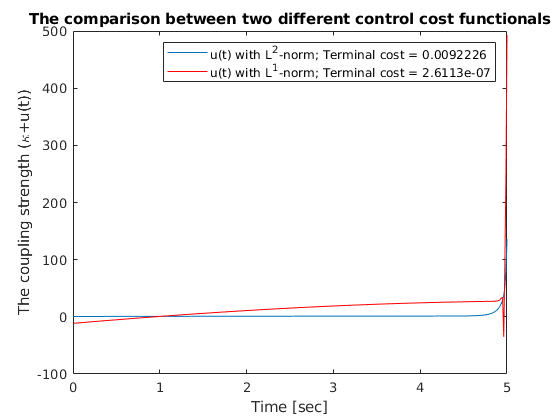The parameter $\omega_i$ and $\kappa$ should be specified for the calculations. Practically, $K > \vert \max\Omega - \min\Omega \vert$ leads to the synchronization of frequencies. We normalize the coupling strength to 1, and give random values for the natural frequencies from the normal distribution $N(0,0.1)$. We also choose initial data from $N(0,pi/4)$.

%% Om_init = normrnd(0,0.1,m,1);
%% Om_init = Om_init - mean(Om_init);  %% Mean zero frequencies
%% Th_init = normrnd(0,pi()/4,m,1);

K_init = ones(m,m);                 %% Constant coupling strength, 1.
T = 5;                              %% We give enough time for the frequency synchronization.

path_data = replace(which(file),file,'');

symF = subs(Vsys,[symOm,symK],[Om_init,K_init]);
Params = sym.empty;
symFFcn = matlabFunction(symF,'Vars',{t,symTh,symU,Params});
odeEqn = ode(symFFcn,symTh,symU,'InitialCondition',Th_init,'FinalTime',T,'Nt',400);


We next construct cost functional for the control problem.

symPsi = @(T,symThth)      10000*norm(sin(symThth.' - symThth),'fro');   %% Sine distance for the periodic interval $[0,2pi]$.
symL_1 = @(t,symThth,symU) (symU.'*symU);                    %% Set the L^2 regularization for the control $u(t)$.
%%
iCP_1 = Pontryagin(odeEqn,symPsi,symL_1);
%%
U0 = zeros(length(iCP_1.Dynamics.tspan),iCP_1.Dynamics.ControlDimension);


tic
toc


Solve with precision:

We obtain: J(u) = 2.612700E+02

error = 2.330901E+00

With 94 iterations,     In 5.9585 seconds

Elapsed time is 5.959747 seconds.



## Visualization

First, we present the dynamics without control,

[tspan, ThetaVector] = solve(odeEqn);
clf
plot(tspan',ThetaVector)
legend("\theta_"+[1:m])
xlabel('Time [sec]')
title('The dynamics without control (incoherence)')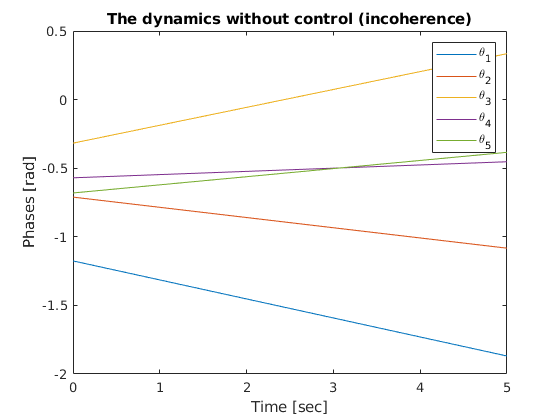and see the controled dynamics.

odec_1 = iCP_1.Dynamics;
clf
plot(odec_1.tspan',odec_1.StateVector.Numeric(:,:))
legend("\theta_"+[1:m])
xlabel('Time [sec]')
title('The dynamics under control')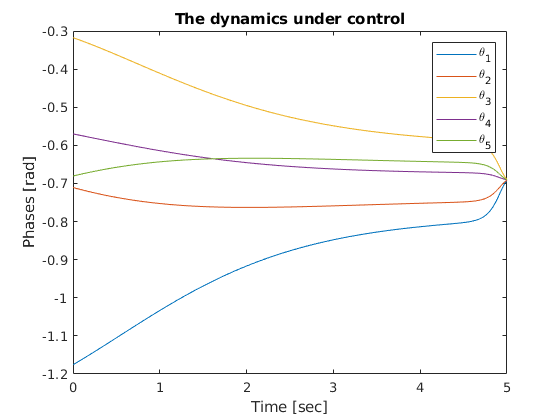We also can plot the control function along time.

clf
Ufinal_1 = iCP_1.Solution.UOptimal;
plot(odec_1.tspan',Ufinal_1)
legend("norm(u(t)) = "+norm(Ufinal_1))
ylabel('u(t)')
xlabel('Time [sec]')
title('The control function')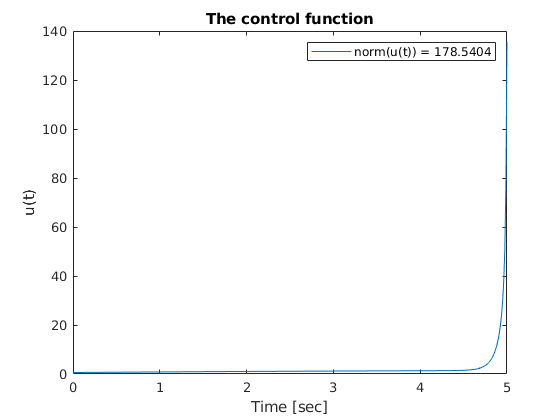## The problem with different regularization

In this part, we change the regularization into L^1-norm and see the difference.

symL_2 = @(t,Y,symU)   abs(symU);
iCP_2 = Pontryagin(odeEqn,symPsi,symL_2);
%%
tic
toc


Solve with precision:

We obtain: J(u) = 7.808495E+01

error = 9.356892E-01

With 6 iterations,     In 1.0928 seconds

Elapsed time is 1.093697 seconds.


odec_2 = iCP_2.Dynamics;
clf
plot(odec_2.tspan',odec_2.StateVector.Numeric(:,:))
legend("\theta_"+[1:m])
xlabel('Time [sec]')
title('The dynamics under control with different regularization')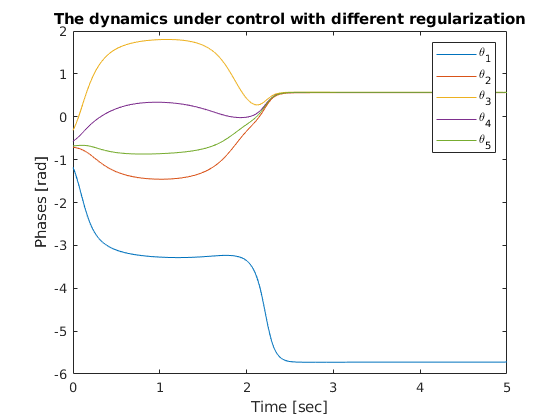Ufinal_2 = iCP_2.Solution.UOptimal;
figure
plot(odec_1.tspan',Ufinal_1)
line(odec_2.tspan',Ufinal_2,'Color','red')

Thfinal_1 = odec_1.StateVector.Numeric(end,:);
Thfinal_2 = odec_2.StateVector.Numeric(end,:);
Psi_1 = norm(sin(Thfinal_1.' - Thfinal_1),'fro');
Psi_2 = norm(sin(Thfinal_2.' - Thfinal_2),'fro');

legend("u(t) with L^2-norm; Terminal cost = "+Psi_1,"u(t) with L^1-norm; Terminal cost = "+Psi_2)
ylabel('The coupling strength (\kappa+u(t))')
xlabel('Time [sec]')
title('The comparison between two different control cost functionals')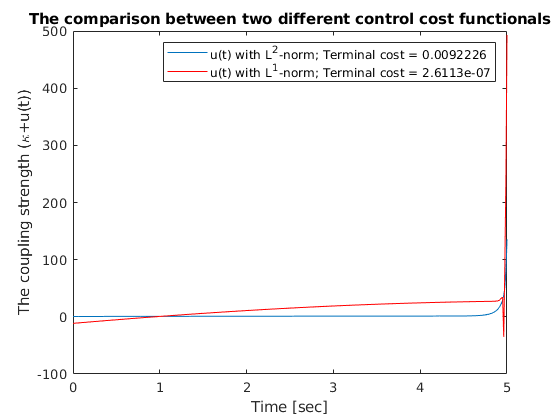As one can expected from the regularization functions, the control function from $L^2$-norm acting more smoothly from 0 to the largest value. The function from $L^2$-norm draws much stiff lines.

YFr = odeEqn.StateVector.Numeric;
YL1 = iCP_1.Dynamics.StateVector.Numeric;
YL2 = iCP_2.Dynamics.StateVector.Numeric;
%%
%% animationpendulums({YFr,YL1,YL2},tspan,{'Free','L^2 Control','L^1 Control'})